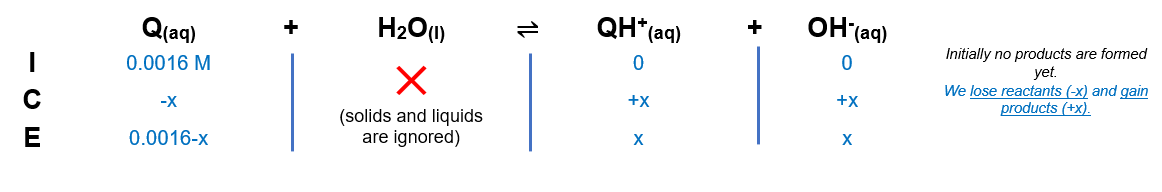# Problem: Quinine (C20H24N2O2) is the most important alkaloid derived from cinchona bark. It is used as an antimalarial drug. For quinine, pK b1 = 5.1 and pK b2 = 9.7 (pK b = -log K b). Only 1 g quinine will dissolve in 1900.0 mL of solution. Calculate the pH of a saturated aqueous solution of quinine. Consider only the reaction Q + H2O ⇌ QH + + OH - described by pK b1, where Q = quinine.

###### FREE Expert Solution

We are asked to calculate the pH of a saturated aqueous solution of quinine.

Step 1. Calculate concentration

Molar mass = 324.4 g/mol

Molarity = 0.0016 M

Step 2. Set-up ICE chartStep 3. Calculate [OH-]

$\overline{){{\mathbf{K}}}_{{\mathbf{b}}}{\mathbf{=}}\frac{\mathbf{\left[}{\mathbf{QH}}^{\mathbf{+}}\mathbf{\right]}\mathbf{\left[}{\mathbf{OH}}^{\mathbf{-}}\mathbf{\right]}}{\left[Q\right]}}\phantom{\rule{0ex}{0ex}}{\mathbf{10}}^{\mathbf{-}{\mathbf{pK}}_{\mathbf{b}}}\mathbf{=}\frac{\mathbf{\left[}\mathbf{x}\mathbf{\right]}\mathbf{\left[}\mathbf{x}\mathbf{\right]}}{\left[0.0016-x\right]}\phantom{\rule{0ex}{0ex}}{\mathbf{10}}^{\mathbf{-}\mathbf{5}\mathbf{.}\mathbf{1}}\mathbf{=}\frac{\mathbf{\left[}\mathbf{x}\mathbf{\right]}\mathbf{\left[}\mathbf{x}\mathbf{\right]}}{\left[0.0016-x\right]}\phantom{\rule{0ex}{0ex}}\mathbf{7}\mathbf{.}\mathbf{94}\mathbf{×}{\mathbf{10}}^{\mathbf{-}\mathbf{6}}\mathbf{=}\frac{\mathbf{\left[}\mathbf{x}\mathbf{\right]}\mathbf{\left[}\mathbf{x}\mathbf{\right]}}{\left[0.0016-x\right]}$

Check if x in the denominator is negligible:

Since the ratio is < 500, x is not negligible.

From the equation, we have a = 1, b = 7.94×10–6, and c = 1.27 × 10–8. Solving for x

[OH-] = x = 1.09 x 10-4 M###### Problem Details

Quinine (C20H24N2O2) is the most important alkaloid derived from cinchona bark. It is used as an antimalarial drug. For quinine, pK b1 = 5.1 and pK b2 = 9.7 (pK b = -log K b). Only 1 g quinine will dissolve in 1900.0 mL of solution. Calculate the pH of a saturated aqueous solution of quinine. Consider only the reaction Q + H2O ⇌ QH + + OH - described by pK b1, where Q = quinine.